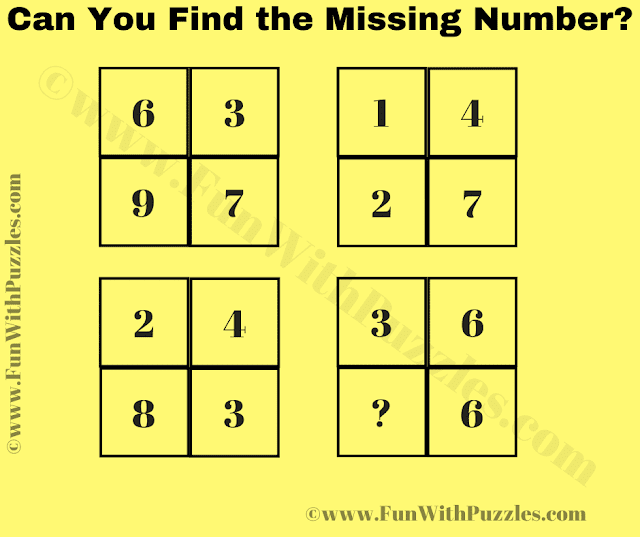This Logic Maths Puzzle Question is for school students. This is the test of your mathematical skills and logical reasoning. Teachers can use this puzzle to ask their students to find the missing number to test their student's maths and logical abilities. Anyway, in this puzzle question, your challenge is to crack the hidden math logic code in this puzzle which relates to the given numbers in the Square.  Can you crack the code and find the value of the missing number in this puzzle?Can you Find the Missing Number?

The answer to this "Logic Maths Puzzle Question for Students", can be viewed by clicking on the answer button.